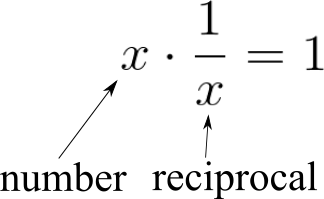# Reciprocal Calculator

Created by Hanna Pamuła, PhD candidate
Reviewed by Steven Wooding and Jack Bowater
Last updated: Jan 07, 2022

If you're wondering how to find the reciprocal, we're here to help with this easy-to-use reciprocal calculator.
Below, you can find an explanation of what a reciprocal is and examples of how to calculate and find the reciprocals, be it the reciprocal of a fraction or a number.

As you're interested in reciprocals, we suspect that fraction calculators may be interesting to you as well. So why not check out our other tools!

## What is a reciprocal?

A reciprocal in math is one divided by the number in question (also known as the multiplicative inverse).

The reciprocal of x = 1/x

Alternatively, you can say that if you multiply a number by its reciprocal, you'll get 1:For example, if our chosen number is 5, then its reciprocal is 1/5. The multiplication of these two numbers will give us 1:

• 5 * 1/5 = 5 * 0.2 = 1

The name reciprocal comes from Latin, possibly from the phrase reque proque meaning back and forth. The reciprocal number to x may be denoted simply as 1/x, but also as x-1. Thus, raising the number to the power of minus one is the same as finding its reciprocal.

## How to find the reciprocal?

So, in short, how to find the reciprocal of a number?

1. Reciprocal of a fraction

To find the reciprocal of a fraction, switch the numerator and the denominator (the top and bottom of the fraction, respectively). So, simply speaking, the reciprocal of a/b is b/a.

Example: the reciprocal of 3/4 is 4/3

2. Reciprocal of a number

To find the reciprocal of a number, divide 1 by the number.

Example: the reciprocal of 7 is 1/7

3. Reciprocal of a decimal

To find the reciprocal of a decimal, you need to do the same as before - divide 1 by your decimal number.

Example: the reciprocal of 3.25 is 1/3.25

Additionally, our reciprocal calculator will show you the fraction in its simplified form.

Remember that 0 doesn't have the reciprocal, as 1/0 is undefined.

## Examples: what is the reciprocal of 4 and 1/2?

We hope that, after our explanation, you now understand the concept of a reciprocal. In that case, let's look at two examples to check how this reciprocal calculator works!

Example 1: What is the reciprocal of 4?

• Is your number a fraction? Not this time! Choose No from the drop-down list. (If you really want, you can leave it as the fraction option and then choose 1 as the denominator).
• Input the number for which you'd like to find the reciprocal, which is 4.
• Tadaa! That's all you need to do! The reciprocal calculator shows that the reciprocal of your number is 0.25, or, as a fraction, 1/4.

Example 2: What is the reciprocal of 1/2?

• Again, start with the question is the number is a fraction? Pick Yes this time.
• Enter the numerator (top) and denominator (bottom) of your fraction into the appropriate fields. So, in our case, input 1 as the numerator and 2 in the denominator box.
• The reciprocal calculator finds the answer - the reciprocal of 1/2 is 2.

## FAQ

### What is the reciprocal of 5?

0.2. Since we can write 5 as 5/1, if we take the reciprocal, it becomes 1/5, which is 0.2 in decimal form.

### What is the reciprocal of 2/3?

3/2 or 1.5. Taking the reciprocal of a fraction means swapping the numerator and the denominator around.

### What is the reciprocal of 1?

1. Since 1 in fractional form is written as 1/1, taking the reciprocal (swapping around the numerator and the dominator) results in the same thing, 1/1, which is 1.

### How do you find the inverse of a fraction?

You find the inverse of a fraction by exchanging the numerator (top number) with the denominator (bottom number) – taking the reciprocal of the fraction. For example, the inversion of the fraction 5/4 is 4/5.

Hanna Pamuła, PhD candidate
Input in the form of
an integer/decimal
Number
Result
Reciprocal
People also viewed…

### Discount

Discount calculator uses a product's original price and discount percentage to find the final price and the amount you save.

### Eggs

Quantum physicist's take on boiling a perfect egg.

### Graphing quadratic inequalities

Graphing quadratic inequalities calculator will show you how to solve your homework problems by drawing plots rather than performing daunting calculations!

### Perimeter of a rectangle

How to find the perimeter of a rectangle? What is the perimeter of a rectangle formula? Check out this perimeter of a rectangle calculator and quickly estimate the necessary parameters of a rectangle.